# Modeling Fluid flows in ThermoCycle

The finite volume approach is selected as general method to simulate fluid flow in the library. The basic fluid flow component is a cell in which the energy and mass conservation equations are applied.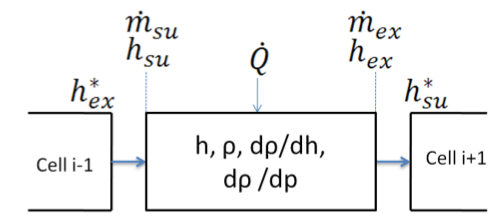Two types of variables can be identified: cell variables and node variables. Node variables are distinguished by the su (supply) and ex (exhaust) subscripts, and correspond to the inlet and outlet nodes of each cell. The relation between the cell and node values depends on the discretization scheme. In the cell component two schemes are implemented, the central difference scheme ( h = (h_su + h_ex)/2 ) and the upwind scheme ( h=h_su ). Since the model accounts for flow reversal, a conditional statement is added depending on the flow rates at the inlet and outlet nodes. For the central difference scheme, h_su is expressed by the following equation (an equivalent equation applies to h_ex):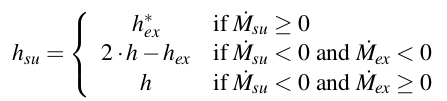where the flow rates are defined as positive when the fluid flows in the nominal direction (from su to ex), and where h_ex indicates the exhaust node enthalpy of the previous cell. For the upwind scheme: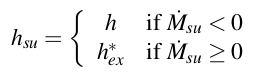The energy balance is expressed by: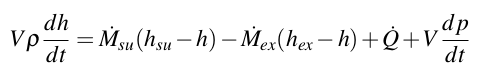The mass balance is expressed by: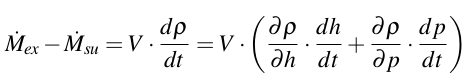The cell model has been developed for compressible, incompressible, and constant heat capacity fluid. The overall flow model can be obtained by connecting several cells in series. The final discretization scheme corresponds to a staggered grid.